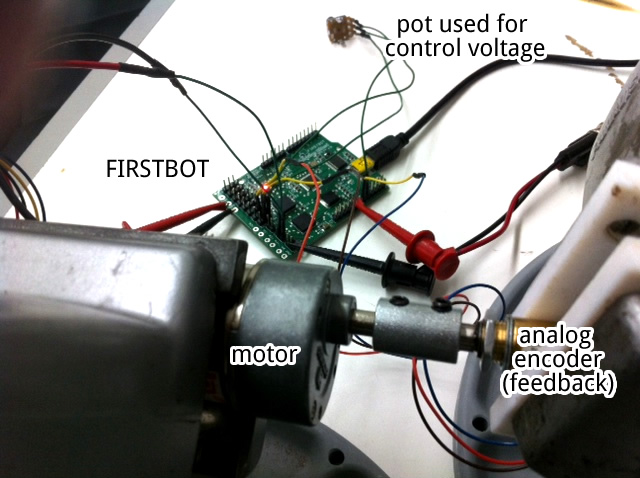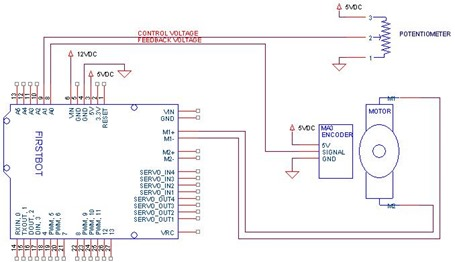Loading... Please wait...

# PID Motor Control with an Arduino

Posted byPID motor control with an Arduino can be accomplished using simple firmware.  In this example we use our Firstbot Arduino-Compatible controller to implement a PID based position controller using analog feedback and a potentiometer for control.  This is similar in operation to a hobby servo, but the potentiometer provides the control signal instead of a pulse from a receiver (and of course you are using a motor, not an RC servo).PID control is fairly common means of controlling a system using a well defined algorithm.  You can learn more about the algorithm at Wikipedia ( see PID Controller). They are used in industrial control systems of all types.  In this case I’m using it to control the position of a motor.  I have a potentiometer that outputs a 0-5VDC control voltage.  Attached to my motor shaft is an analog encoder that also outputs a value of 0-5VDC over a single rotation of the motor (US Digital MA3 encoder).   The idea is that when I turn the pot the motor follows the turn and stops where the pot stops.

P, I, and D stand for proportional, integral, and derivative.  Generally speaking the proportional (P) part of the control algorithm provides most of the “push” to get things moving.  The integral (I) term is used to act on small errors and force gradual changes over time.  The derivative (D) part of the equation acts to damp oscillations or abrupt changes in the control signal.  The D term is usually works to oppose abrupt changes caused by the P term.

Here’s the code for the equation I used.  The gain constants are set in the code, but could be made programmable through some other interface.

```void CalculatePID(void)
{
// Set constants here
PTerm = 2000;
ITerm = 25;
DTerm = 0;
Divider = 10;

// Calculate the PID
Accumulator += Error;  // accumulator is sum of errors
PID += ITerm*Accumulator; // add integral gain and error accumulation
PID += DTerm*(Error-Error); // differential gain comes next```

Here are the major components of the PID detailed.

Error Signal-  At the heart of PID control is a need to measure an error signal.  In this case it is the desired position (voltage from the pot) minus the actual position (voltage from the encoder).  The error value is signed.

```void GetError(void)
{
byte i = 0;

// shift error values
for(i=9;i>0;i--)
Error[i] = Error[i-1];

// load new error into top array spot
Error = (long)DesiredPosition-(long)ActualPosition;

}```

PTerm –The error signal is multiplied the P term and this provides most of the “umph” behind the motor’s movement.  A negative error signal causes the P term create a negative motor movement.  Likewise, if my error signal is 0 then the P term has no impact on the motor.

`PID = Error*PTerm;     // start with proportional gain`

ITerm – The I term is generally much smaller than the P term.  There is also an accumulator associated with the I term.  The accumulator sums up error signals over time.  Eventually even small errors build up to be large number, when that happens a small I term will  cause to move the motor.

```Accumulator += Error;  // accumulator is sum of errors
PID += ITerm*Accumulator; // add integral gain and error accumulation```

In some systems it is important to prevent “windup” of the accumulator(also called saturation).  Windup occurs when the small errors build so high that when movement finally occurs it takes a long time for the accumulator to reduce to an insignificant amount.  I didn’t add any windup protection here, but it can often be addressed by limiting the size of the accumulator.

Example:

if (Accumulator > 40000)

Accumulator = 40000;

if (Accumulator < -40000)

Accumulator = -40000;

DTerm – While I have the D term in this equation it is not used for this motor control application (see DTerm = 0 in the code).  The D term is multiplied by the change in the error signal (error – last error).  Sometimes it is useful to store your error measurements in an array and use as your last error something a little further back in time.  For fast changing systems, like a motor controller, the derivative portion of the PID has little impact unless you make it very large, or compare error signals with adequate time between their sampling.  In this code the derivative error is the latest error signal minus the 10th previous error.

`PID += DTerm*(Error-Error); // differential gain comes next`

Divider - When you put the PID together you get a pretty big value.  This value needs to be scaled to a value that matched the pulse-width modulation range for the controller.  The Divider does that.  You’ll notice that the division of the PID is accomplished by right-rotates as opposed to division.  This is just a faster way of accomplishing the same thing.  And the faster your PID loop runs the more responsive it can be to commanded changes.

`PID = PID>>Divider; // scale PID down with divider`

Converting the PID to PWM- Once your PID is calculated you need to change it to a motor drive signal.  The sign of the PID output determines the direction the motor should be driven and the divider previously discussed should get you in the right neighborhood for a final number.  Now you need to make sure the PID register contains a value that’s neither too large or too small.

```if(PID>=127)
PID = 127;
if(PID<=-126)
PID = -126;

//PWM output should be between 1 and 254 so we add to the PID
PWMOutput = PID + 127;```

The FIRSTBOT accepts a range of 1 to 254 (1 = full reverse, 127 = stopped, 254 = full forward).  So we want our PID to be in the area of –126 to +127, and we’ll add 127 to it to get our 1-254 range.

Tuning the PID- Trail and error works.  There are a number of methods of tuning PID algorithms, you can research those online.  For something like a generic motor position controller using analog signals you can start by adjusting your proportional settings until you get rough control.  If your proportional term is too high the movement will be sharp and choppy.  If too low, it will be slow.  This is also when you dial in the divider value to make sure your PID output falls within your PWM requirements.

Then increase your integral term until the final desired position is reached.  The integral term is usually much smaller than the proportional term.  An integral term that is too high will cause oscillations of the motor.  Too low and it has no impact at all.  If your motor tends to twitch you may need to add some kind of windup detection as discussed above.

The settings I used for this design got me to within +/-10 ADC counts within a couple of seconds.  That is right at about 1% accuracy or 3.5 degrees for a single rotation.

The code has a lot of commented out print statements that can be commented in during certain tests to see what those variables look like.  Those can be useful when tuning the PID.

PID Loop Time:  You can see from this code that it is very simple.  The serial communication that sends data to the FIRSTBOT’s other motor controller IC (a PIC16F1829) occurs every 10mS.  Not super fast, but it certainly works for many applications.

Here’s all of the code together.

```/*
Firstbot PID code:  Implements a PID controller using

The circuit:
* RX is digital pin 2 (connect to TX of other device)
* TX is digital pin 3 (connect to RX of other device)

*/
#include <SoftwareSerial.h>

// define some constants
int ActPos = A0;    // select the input pin for feedback signal
int DesPos = A1;    // select the input pin for control signal

byte PWMOutput;
long Error;
long Accumulator;
long PID;
int PTerm;
int ITerm;
int DTerm;
byte Divider;

/*
The FIRSTBOT has a PIC16F1829 controller that controls the
two MC33926 H-bridges on the board.  A oftware serial interface
is used to control that part.
*/
SoftwareSerial mySerial(2, 3); // Receive data on 2, send data on 3
byte SerialTXBuffer;
byte SerialRXBuffer;

void setup()
{

// Open serial communications and wait for port to open:
Serial.begin(9600);
mySerial.begin(9600);
}

/* GetError():
Read the analog values, shift the Error array down
one spot, and load the new error value into the
top of array.
*/
void GetError(void)
{
byte i = 0;
// comment out to speed up PID loop
//  Serial.print("ActPos= ");
//  Serial.println(ActualPosition,DEC);

// comment out to speed up PID loop
//  Serial.print("DesPos= ");
//  Serial.println(DesiredPosition,DEC);

// shift error values
for(i=9;i>0;i--)
Error[i] = Error[i-1];
// load new error into top array spot
Error = (long)DesiredPosition-(long)ActualPosition;
// comment out to speed up PID loop
//  Serial.print("Error= ");
//  Serial.println(Error,DEC);

}

/* CalculatePID():
Error is used for latest error, Error with the DTERM
*/
void CalculatePID(void)
{
// Set constants here
PTerm = 2000;
ITerm = 25;
DTerm = 0;
Divider = 10;

// Calculate the PID
Accumulator += Error;  // accumulator is sum of errors
PID += ITerm*Accumulator; // add integral gain and error accumulation
PID += DTerm*(Error-Error); // differential gain comes next
PID = PID>>Divider; // scale PID down with divider

// comment out to speed up PID loop
//Serial.print("PID= ");
//  Serial.println(PID,DEC);

// limit the PID to the resolution we have for the PWM variable

if(PID>=127)
PID = 127;
if(PID<=-126)
PID = -126;

//PWM output should be between 1 and 254 so we add to the PID
PWMOutput = PID + 127;

// comment out to speed up PID loop
//  Serial.print("PWMOutput= ");
//  Serial.println(PWMOutput,DEC);

}

/* WriteRegister():
Writes a single byte to the PIC16F1829,
"Value" to the register pointed at by "Index".
Returns the response
*/
byte WriteRegister(byte Index, byte Value)
{
byte i = 0;
byte checksum = 0;
byte ack = 0;

SerialTXBuffer = 210;
SerialTXBuffer = 1;
SerialTXBuffer = 3;
SerialTXBuffer = Index;
SerialTXBuffer = Value;

for (i=0;i<6;i++)
{
if (i!=5)
{
mySerial.write(SerialTXBuffer[i]);
checksum += SerialTXBuffer[i];
}
else
mySerial.write(checksum);
}
delay(5);

if (mySerial.available())

return ack;
}

void loop() // run over and over
{
GetError();       // Get position error
CalculatePID();   // Calculate the PID output from the error
WriteRegister(9,PWMOutput);  // Set motor speed
}```